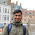## November 11, 2012

### All paths from source to destination in simple graph

UPDATE: I've written a new tutorial for this question with more explanation and comments in code. I highly recommend you to go through this new link for better understanding.

https://techienotes.info/2015/09/03/all-paths-between-two-nodes-in-a-graph/

Q: Given a simple graph, print all the paths from source node to destination node.

A: We're going to traverse the graph and try all the possible paths we could get. We'll start DFS(Depth First Search) from the source node and will keep looking the different paths to destination during the course of complete dfs() procedure.
Input is given as 2-D matrix where 1 represents an edge and 0 represents no edge. In output there will be a path printed per line.

Here's the Java code for the same.
```import java.io.BufferedReader;
import java.io.FileReader;
import java.io.IOException;
import java.util.ArrayList;
import java.util.Arrays;
import java.io.InputStreamReader;

class allpaths {

static int dim = 5, size = 0; // dim is number of nodes in graph
// size had been used to keep record of index to remove node from Arraylist
static boolean[] color = new boolean[dim + 1];      // to remember visit
static int[][] graph = new int;
static ArrayList<Integer> al = new ArrayList<Integer>();

public static void main(String[] S) throws IOException {
BufferedReader br = new BufferedReader (new InputStreamReader(System.in));
for (int I = 1; I <= dim; I++) {
String[] s = br.readLine().split(" ");
int len = s.length;
for (int J = 1; J <= len; J++) {
graph[I][J] = Integer.parseInt(s[J - 1]);
}
}
Arrays.fill(color, false);      // initially all are unvisited

int src = Integer.parseInt(br.readLine());      //Source node
int dst = Integer.parseInt(br.readLine());      //Destination node

dfs(src, dst);  // backtracking
}

static void dfs(int src, int dst) {
al.add(src);
size++;
color[src] = true;
if (src == dst) {       // tests for base condition to stop
for (Integer i : al) {
//     Prints the path
System.out.print(i + "  ");
}
System.out.println();
return;
}
for (int I = 1; I <= dim; I++) {
if (graph[src][I] == 1) {
if (color[I] == false) {
dfs(I, dst);        // These lines do
color[I] = false;   // main job of backtracking
size--;
al.remove(size);
}
}
}
}
}```
`Input is given as`
```0 1 1 1 1
1 0 1 1 1
1 1 0 1 1
1 1 1 0 1
1 1 1 1 0
1
5
```

This is complete graph for 5 vertices. Source node is 1 and Destination node is 5. Output would look like this:

1  2  3  4  5
1  2  3  5
1  2  4  3  5
1  2  4  5
1  2  5
1  3  2  4  5
1  3  2  5
1  3  4  2  5
1  3  4  5
1  3  5
1  4  2  3  5
1  4  2  5
1  4  3  2  5
1  4  3  5
1  4  5
1  5

Here each line shows a path from 1 to 5 via other nodes.

#### 1 comment:

1.Thanx a lot man this code of yours saved me. God bless you. :)